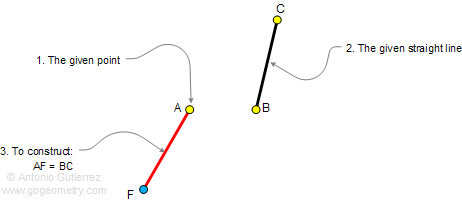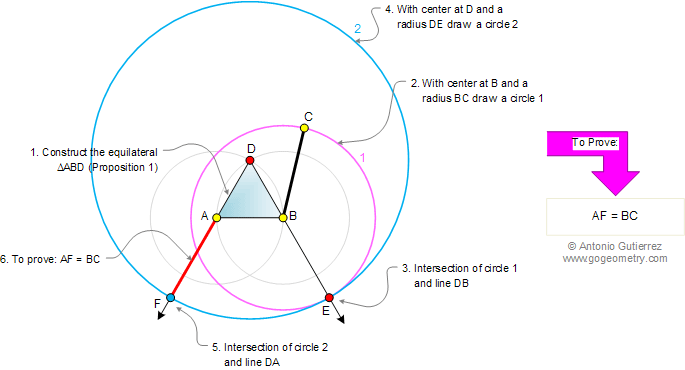Euclid's Elements Book I, Proposition 2: To place at a given point (as an extremity) a straight line equal to a given straight line

Let A be the given point, and BC the given straight line. Thus it is required to place at the point A (as an extremity) a straight line equal to the given straight line BC.ConstructionThe Elements: Books I-XIII

 Euclid's Elements is the oldest mathematical and geometric treatise consisting of 13 books written by Euclid in Alexandria c. 300 BC. It is a collection of definitions, postulates, axioms, 467 propositions (theorems and constructions), and mathematical proofs of the propositions.